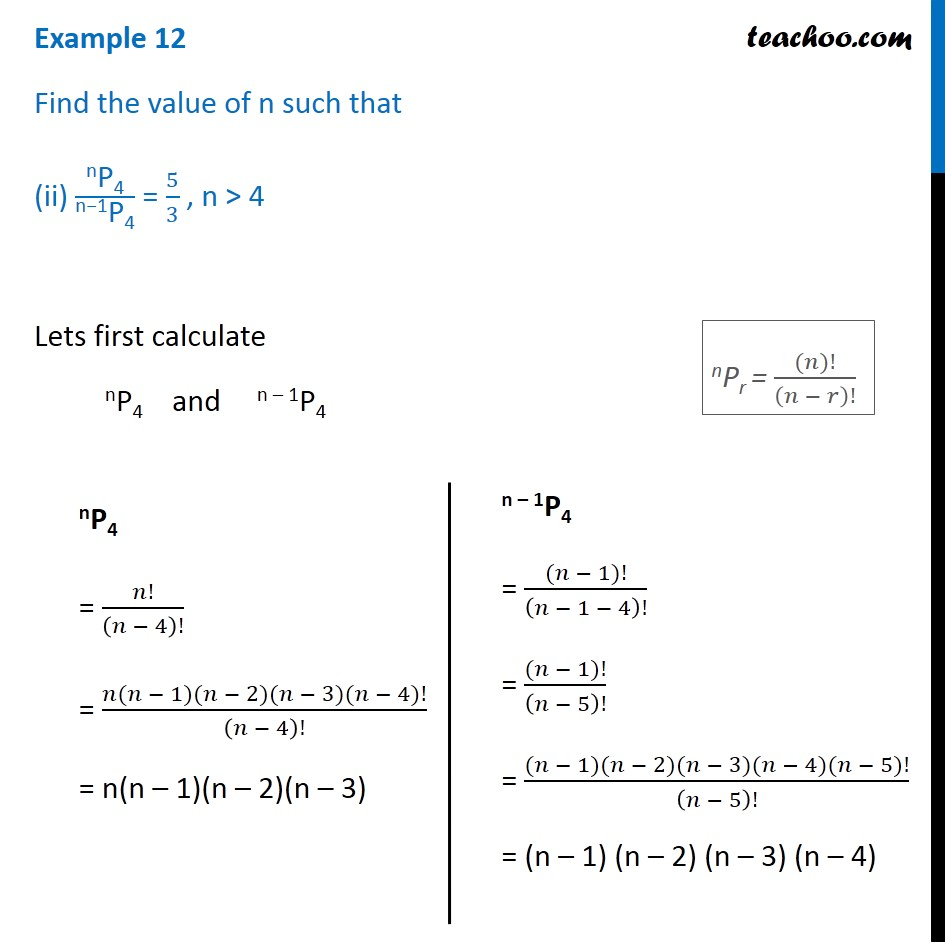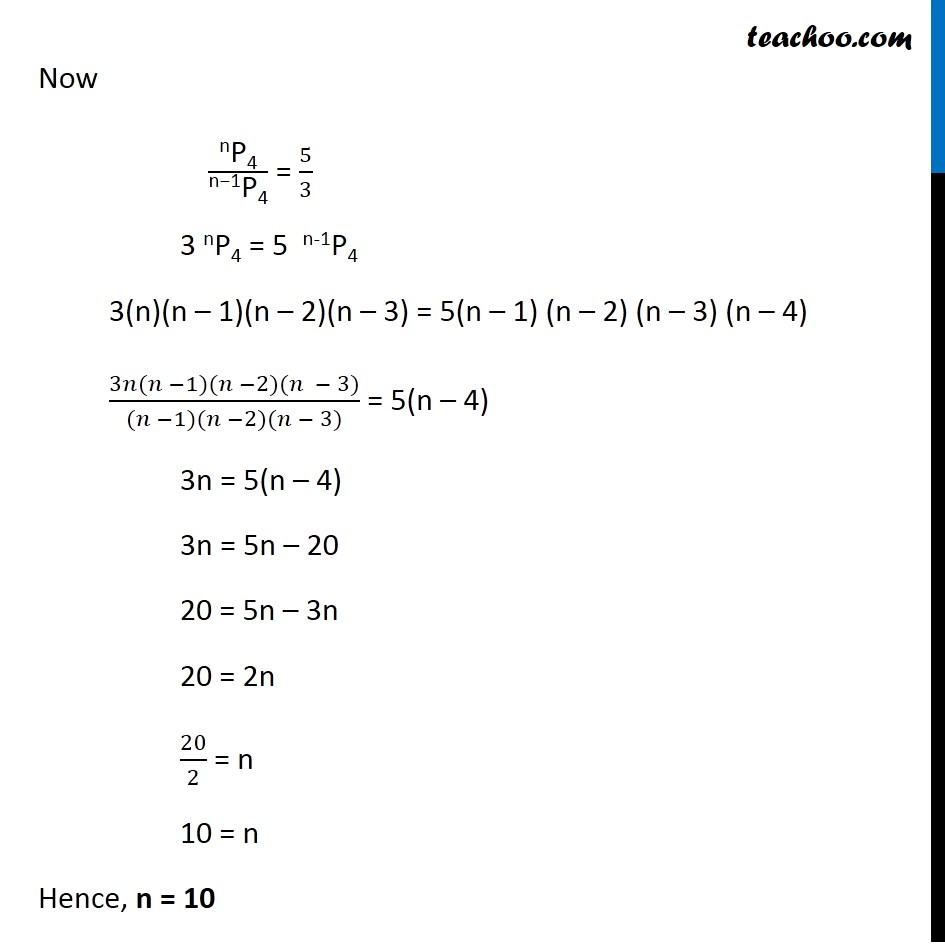Examples

Chapter 6 Class 11 Permutations and Combinations
Serial order wiseLearn in your speed, with individual attention - Teachoo Maths 1-on-1 Class

### Transcript

Example 12 Find the value of n such that (ii) "nP4" /"n−1P4" = 5/3 , n > 4 Lets first calculate nP4 and n – 1P4 nP4 = 𝑛!/(𝑛 − 4)! = (𝑛(𝑛 − 1)(𝑛 − 2)(𝑛 − 3)(𝑛 − 4)!)/(𝑛 − 4)! = n(n – 1)(n – 2)(n – 3) n – 1P4 = ((𝑛 − 1)!)/(𝑛 − 1 − 4)! = ((𝑛 − 1)!)/(𝑛 − 5)! = ((𝑛 − 1)(𝑛 − 2)(𝑛 − 3)(𝑛 − 4)(𝑛 − 5)!)/(𝑛 − 5)! = (n – 1) (n – 2) (n – 3) (n – 4) nPr = ((𝑛)!)/(𝑛 − 𝑟)! Now "nP4" /"n−1P4" = 5/3 3 nP4 = 5 n-1P4 3(n)(n – 1)(n – 2)(n – 3) = 5(n – 1) (n – 2) (n – 3) (n – 4) (3𝑛(𝑛 −1)(𝑛 −2)(𝑛 − 3))/((𝑛 −1)(𝑛 −2)(𝑛 − 3)) = 5(n – 4) 3n = 5(n – 4) 3n = 5n – 20 20 = 5n – 3n 20 = 2n 20/2 = n 10 = n Hence, n = 10 n(n – 10) + 3(n – 10) = 0 (n – 10) (n + 3) = 0 So, n = 10, and n = –3 But, It is given in question n > 4 So n = –3 not possible Therefore, n = 10 only Example 12 Find the value of n such that (ii) "nP4" /"n−1P4" = 5/3 , n > 4 Lets first calculate nP4 and n – 1P4 nP4 = 𝑛!/(𝑛 − 4)! = (𝑛(𝑛 − 1)(𝑛 − 2)(𝑛 − 3)(𝑛 − 4)!)/(𝑛 − 4)! = n(n – 1)(n – 2)(n – 3) n – 1P4 = ((𝑛 − 1)!)/(𝑛 − 1 − 4)! = ((𝑛 − 1)!)/(𝑛 − 5)! = ((𝑛 − 1)(𝑛 − 2)(𝑛 − 3)(𝑛 − 4)(𝑛 − 5)!)/(𝑛 − 5)! = (n – 1) (n – 2) (n – 3) (n – 4) nPr = ((𝑛)!)/(𝑛 − 𝑟)! Now "nP4" /"n−1P4" = 5/3 3 nP4 = 5 n-1P4 3(n)(n – 1)(n – 2)(n – 3) = 5(n – 1) (n – 2) (n – 3) (n – 4) (3𝑛(𝑛 −1)(𝑛 −2)(𝑛 − 3))/((𝑛 −1)(𝑛 −2)(𝑛 − 3)) = 5(n – 4) 3n = 5(n – 4) 3n = 5n – 20 20 = 5n – 3n 20 = 2n 20/2 = n 10 = n Hence, n = 10